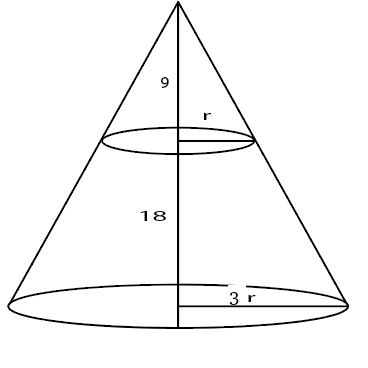Question 6

# A solid right circular cone of height 27 cm is cut into two pieces along a plane parallel to its base at a height of 18 cm from the base. If the difference in volume of the two pieces is 225 cc, the volume, in cc, of the original cone is

SolutionLet the base radius be 3r.

Height of upper cone is 9 so, by symmetry radius of upper cone will be r.

Volume of frustum=$$\frac{\pi}{3}\left(9r^2\cdot27-r^2.9\right)$$

Volume of upper cone = $$\frac{\pi}{3}.r^2.9$$

Difference= $$\frac{\pi}{3}\cdot9\cdot r^2\cdot25=225$$ => $$\frac{\pi}{3}\cdot r^2=1$$

Volume of larger cone = $$\frac{\pi}{3}\cdot9r^2\cdot27=243$$

OR